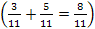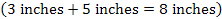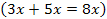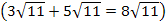# Fractions - What is one-half plus one-third?

## Review Sheet - Yellow Pages

• This is a comprehensive review of fractions, decimals, and percents

## Online Practice

• Fraction Quiz - Test your fraction skills, including decimals and percentages. You may choose difficulty level, question types, and time limit. (Similar format to Arithmetic Four* above.) Five type of questions to choose from: (1) Fraction asks you to +, -, x, ÷, fractions and mixed numbers, and determine which quantity is smaller; (2) Percentage has you find a percent as in "16 is what percent of 20" or find percent of a number as in "75% of 28"; (3) Percent<->Decimal has you convert from fractions to decimals and decimals to fractions; (4) Fraction<->Percentage asks you to convert to a percent; (5) Algebra asks you to solve basic algebra questions with fractions (including proportions).
• Fractions at AAA Math - Variety of ways to practice and play games.

## My PFD Page

Percents Fractions and Decimals (PFD) – Rational Numbers and Proportional Reasoning

## Happy Birthday Song

The Happy Birthday Song is a good way to learn and remember to use common denominators. (I'm still fixing the graphics and the Word file...)

"Happy Birthday Song"

Verse 1You must have common denominators,
You must have common denominators,
You must have common denominators.

Verse 2You must have like units,
You must have like units,
You must have like units.

Verse 3You must have like terms,
You must have like terms,
You must have like terms.

Verse 4